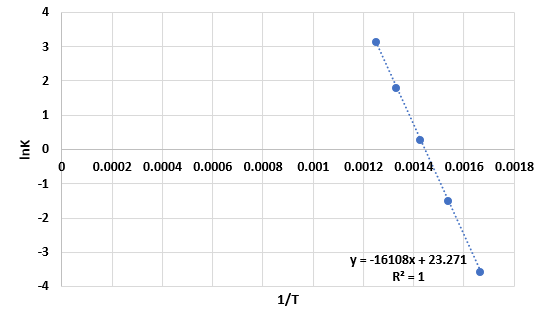# Problem: The temperature dependence of the rate constant for the reaction is tabulated as follows:Temperature (K)   k (M-1s-1) 6000.0286500.227001.37506.080023Calculate A.

###### FREE Expert Solution
83% (432 ratings)
###### FREE Expert Solution

Plot the given data in terms of ln k vs. 1/TThe slope = − 16108 and the intercept = 23.271.

83% (432 ratings)###### Problem Details
The temperature dependence of the rate constant for the reaction is tabulated as follows:

 Temperature (K) k (M-1s-1) 600 0.028 650 0.22 700 1.3 750 6.0 800 23

Calculate A.

What scientific concept do you need to know in order to solve this problem?

Our tutors have indicated that to solve this problem you will need to apply the Arrhenius Equation concept. You can view video lessons to learn Arrhenius Equation. Or if you need more Arrhenius Equation practice, you can also practice Arrhenius Equation practice problems.

What is the difficulty of this problem?

Our tutors rated the difficulty ofThe temperature dependence of the rate constant for the reac...as medium difficulty.

How long does this problem take to solve?

Our expert Chemistry tutor, Sabrina took 11 minutes and 47 seconds to solve this problem. You can follow their steps in the video explanation above.

What professor is this problem relevant for?

Based on our data, we think this problem is relevant for Professor Tai's class at Thomas Jefferson University.